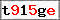This website uses cookies to improve user experience. By using our website you consent to all cookies in accordance with our Cookie Policy. X

### Basics of tile-based engine part 1: isometric view [basic]

In this three-part article I will try to explain some of basic problems one might stumble upon when working with tile-based engines. Since they are quite old one might think that the topic was already exhausted, however many people still have problems with it, often complicating trivial issues.
One of those issues is isometric view (sometimes might be called "diagonal view") which a specific type of 3D transformation without the perspective and was often used in older strategy games. Although the definition mentions 3D transformation it is actually not required at all and can be replaced with something much simpler, which unfortunately not many people try to do, resulting in many articles like this one focusing on basics of 3D - unnecessarily.
Basically you could sum everything to this simple function:
```function getTile(nTargetX:Number,nTargetY:Number,nWidth:Number,nHeight:Number):Point {
var nScale:Number = nWidth/nHeight;
var nTransY:Number = (nScale*nTargetY-nTargetX)/2;
var nTransX:Number = nTargetX+nTransY;
var nTmpY:Number = Math.round( nTransY/(nHeight*nScale) );
var nTmpX:Number = Math.round( nTransX/nWidth );
var nTileX:Number = (nTmpX-nTmpY)*nWidth;
var nTileY:Number = (nTmpX+nTmpY)*nHeight;
return new Point(nTileX,nTileY);
}
```
Which results in this:
(Green circle is the Point returned by the getTile function)
Try it yourself: tile_example.zip

That is pretty much it, now the only thing left to do is explain how getTile works step by step:
1.
var nScale:Number = nWidth/nHeight;
Scale will be used during the transformation to realign the difference between height and width (as the algorithm works only in 1:1 scale).
2.
var nTransY:Number = (nScale*nTargetY-nTargetX)/2;
var nTransX:Number = nTargetX+nTransY;

Here is where a point on the screen gets transformed into a point in the isometric view.
Usually when we are talking about an isometric view, we are thinking about a space that is rotated by 45 degrees in such way that movement to the right is not a simple +X, but rather some value of X plus partly also some Y. The example will probably make more sense:

As we can see the movement of the mouse cursor to the right is transformed into a point that is only partially moved to the right, because partially it is also moved upward. Armed with this knowledge we can try and write a general equation - since movement to the right (+X) gives a bit of upward movement (-Y) we can say that Y gets smaller along with X getting bigger, or in other words: Y = -nTargetX. However we have to remember it is not a straightforward transformation x=>y (otherwise it would result in 90 degrees turn), which is why have to divide the equation by 2 (as 90 degrees divided by 2 gives us 45... in a great simplification). Now that we know Y, getting X is quite trivial as we only need to subtract the Y value from target X (so the distance remains the same as the one before the transformation) and since Y is already negative we need to use the addition Y = nTargetX+nTransY;. By the way, you can switch plus and minus signs between each other to achieve a -45 degrees rotation.
3.
var nTmpY:Number = Math.round( nTransY/(nHeight*nScale) );
var nTmpX:Number = Math.round( nTransX/nWidth );
var nTileX:Number = (nTmpX-nTmpY)*nWidth;
var nTileY:Number = (nTmpX+nTmpY)*nHeight;

Here we are translating given point into a tile, by "shrinking" the whole space using width and height values, then discarding any numbers after the comma and finally bringing everything back to "normal size". Because I love examples here is another one: if tile #0 is 20 pixels width, then every pixel between 0 and 20 will belong to it. So now the question is: how to check which tile owns the seventh pixel? Lets take that 7 and divide it by 20, resulting in 0.35. Now we discard everything after the comma and get our tile with id #0. Lets re-try that for pixel 27; 27/20 = 1.35; drop the comma stuff and we get #1, which is correct because as we already know 27 doesn't belong to #0.

Phew, that is it for today. In two weeks I will write about a very simple path finding algorithm you could implement in a tile-based engine.

Name:
Comment:
Confirm the image code: